﻿• 价格透明
• 信息保密
• 进度掌控
• 售后无忧# 面向对象

<1> 导入模块有五种方式
import 模块名
from 模块名 import 功能名
from 模块名 import *
import 模块名 as 别名
from 模块名 import 功能名 as 别名

``````import 模块1,模块2,...  # 导入方式

``````
``````from 模块名 import 函数名1,函数名2....
``````
``````from modname import *
``````

``````os模块
sys模块
math模块
random模块
datetime模块
time模块
calendar模块
hashlib模块
hmac模块
copy模块
uuid模块</uuid>
``````

1.定义类

``````class 类名:
def 方法1(self,参数列表):
pass
def 方法2(self,参数列表):
pass
``````

2.创建实例对象

``````对象变量名 = 类名()
``````
``````class Cat:
"""这是个猫类"""

def eat(self):
print("小猫在吃东西")

def drink(self):
print("小猫在喝水")

tom = Cat()  # 创建了一个Cat对象
tom.eat()
tom.drink()

hello_kitty = Cat()  # 又创建了一个新的Cat对象
hello_kitty.eat()
hello_kitty.drink()
``````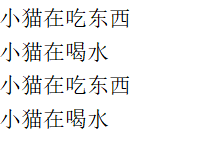3.self的使用

``````tom = Cat()
tom.name = 'Tom'  # 可以直接给 tom 对象添加一个 name 属性
``````
``````class Cat:
def eat(self):
print("%s爱吃鱼" %self.name)

tom = Cat()
tom.name = 'Tom'  # 给 tom 对象添加了一个name属性
tom.eat()  # Tom爱吃鱼

lazy_cat = Cat()
lazy_cat.name = "大懒猫"
lazy_cat.eat() # 大懒猫爱吃鱼
``````"咒语"名字已经由 Python 官方定义好，我们不能乱写。

``````class Student:
def __init__(self, name, age):
self.name = name
self.age = age

def __int__(self):
return self.age

def __float__(self):
return self.age * 1.0

def __str__(self):
return self.name

def __bool__(self):
return self.age > 18

s = Student('zhangsan', 18)
print(int(s))
print(float(s))
print(str(s))
print(bool(s))

if s:
print('hello')
``````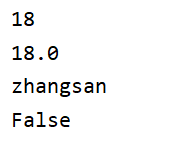``````class Student:
def __init__(self, name, age):
self.name = name
self.age = age

return self.age + other

def __sub__(self, other):
return self.age - other

def __mul__(self, other):
return self.age * other

def __truediv__(self, other):
return self.age / other

def __mod__(self, other):
return self.age % other

def __pow__(self, power, modulo=None):
return self.age ** power

s = Student('zhangsan', 18)
print(s + 1)  # 19
print(s - 2)  # 16
print(s * 2)  # 36
print(s / 5)  # 3.6
print(s % 5)  # 3
print(s ** 2)  # 324
``````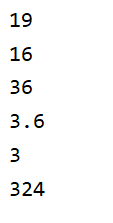``````class Person(object):
def __init__(self,name,age):
# 这里的name和age都属于是实例属性，每个实例在创建时，都有自己的属性
self.name = name
self.age = age

# 每创建一个对象，这个对象就有自己的name和age属性
p1 = Person('张三',18)
p2 = Person("李四",20)
``````

``````
```python
class Dog:
type = "狗"  # 类属性

dog1 = Dog()
dog2 = Dog()

# 不管是dog1、dog2还是Dog类，都可以访问到type属性
print(Dog.type)  # 结果：狗
print(dog1.type)  # 结果：狗
print(dog2.type)  # 结果：狗
``````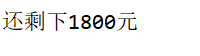``````class Person:
def __init__(self,name,age):
self.name = name
self.age = age
self.__money = 2000  # __money 是私有变量，外部无法访问

def get_money(self):  # 定义了get_money 方法，在这个方法里获取到 __money
return self.__money  # 内部可以访问 __money 变量

def set_money(self,money): # 定义了set_money 方法，在这个方法里，可以修改 __money
self.__money = money

p = Person('王五',21)

# 外部通过调用 get_money 和 set_money 这两个公开方法获取和修改私有变量
print(p.get_money())
p.set_money(8000)
print(p.get_money())
``````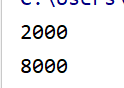``````class Person:
def __init__(self,name,age):
self.name = name
self.age = age
self.__money = 2000

def __shopping(self, cost):
self.__money -= cost

p = Person('李四',20)
print(p._Person__money)  # 使用对象名._类名__私有属性名 可以直接访问对象的私有属性
p._Person__shopping(100)  # 使用对象名._类名__函数名 可以直接调用对象的私有方法
print(p._Person__money)
``````1. 类方法
第一个形参是类对象的方法
需要用装饰器@classmethod来标识其为类方法，对于类方法，第一个参数必须是类对象，一般以cls作为第一个参数。
``````class Dog(object):
__type = "狗"

# 类方法，用classmethod来进行修饰
@classmethod
def get_type(cls):
return cls.__type
print(Dog.get_type())
``````
``````class Dog:

def demo_method(self):
print("对象方法")

@classmethod
def demo_method(cls):
print("类方法")

@staticmethod
def demo_method():  # 被最后定义
print("静态方法")

dog1 = Dog()
Dog.demo_method()  # 结果: 静态方法
dog1.demo_method()  # 结果: 静态方法
````````````class Animal:
def __int__(self):
pass
"""动物类"""
def sleep(self):
print('正在睡觉')

class Dog(Animal):
"""Dog类继承自Animal类"""
def __init__(self):
pass

class Cat(Animal):  # 定义类时，在括号后面传入父类的类名，表示子类继承父类
"""Cat类继承自Animal类"""
def __int__(self):
pass

# Dog 和 Cat 都继承自Animal类，可以直接使用Animal类里的sleep方法
dog = Dog()
dog.sleep()

cat = Cat()
cat.sleep()
``````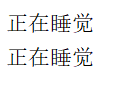``````class Person(object):
def __init__(self, name, age):
self.name = name
self.age = age

p1 = Person('张三', 18)
p2 = Person('张三', 18)
p3 = p1

print(p1 is p2)  # False
print(p1 is p3)  # True
``````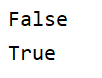isinstance
instance内置函数，用来判断一个实例对象是否是由某一个类(或者它的子类)实例化创建出来的。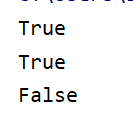issubclass
issubclass 用来判断两个类之间的继承关系。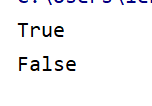``````f = open('test.txt', 'w')
``````
``````# 新建一个文件，文件名为:test.txt
f = open('test.txt', 'w')

# 关闭这个文件
f.close()
``````

``````f = open('test.txt', 'w')
f.write('hello world, i am here!\n' * 5)
f.close()
``````### 低价透明### 金牌服务### 信息保密